Saturday, November 28, 2020
Home > Latest Announcement > NMTC Stage 1 and 2 Sub Junior Level Previous Year Papers with Solutions

# NMTC Stage 1 and 2 Sub Junior Level Previous Year Papers with Solutions# NMTC Stage 1 and 2 Sub Junior Level Previous Year Papers with Solutions

Hi NMTC aspirants, Amans Maths Blogs (AMB) created an ebook of NMTC Previous Year Papers with Solutions according to class wise: for Primary Level (Class 5 & 6), for Sub-Junior Level (Class 7 & 8) and Junior Level (Class 9 & 10). In this post, you will get an ebook of NMTC Stage 1 and 2 Sub Junior Level Previous Year Papers Solutions PDF.

These books are the alternative and best books for NMTC preparation, because previous year questions papers with answer keys and solutions will give an idea of NMTC syllabus and their difficulty level of asked questions in NMTC question paper or NMTC past papers

NMTC is a short form of National Mathematics Talent Competition. This is conducted by AMTI to identify the talent of the students in Mathematics.

Now, for more details about the NMTC exam, like its NMTC eligibility, medium of NMTC papers, examination fee, and NMTC syllabus etc, click here : NMTC Notification

AMTI is a short form of Association Mathematics Teachers of India. It is an academic body of professionals and students who have a passion in Mathematics research and education. For the AMTI NMTC exam, you need amti previous question papers with solutions.

NMTC exams are in two stages. It is called as NMTC Stage 1 and Stage 2 or NMTC Screening Level and NMTC Final Level exam.

To clear NMTC exam, you need NMTC Papers with Solutions it will help you in the preparation of this exam, So I created an EBook of NMTC Previous Question Papers with Solutions PDF.

According to AMTI, the students who have an ability for unique creativity thinking a unique and imaginative considering, preparation to assault new and non-routine issues displaying a general numerical capacity proper to their level. they must start the preparation of NMTC exam.

For the NMTC preparation, you need NMTC study material, or you can also say it as AMTI exam books for NMTC.

Get EBook PDF of NMTC Stage 1 and 2 Primary Level Papers with Solutions

## NMTC Stage 1 Sub Junior Level Previous Years Questions Papers with Solutions

NMTC Sub Junior Level exam is known as Kaprekar Contest, its eligibility is for Class 7 and 8. Thus, to prepare for NMTC Sub Junior level exam, you need NMTC Stage 1 Sub Junior Level Previous Years Questions Papers with Solutions.

### NMTC 2004 Stage 1 Sub Junior Level Questions Paper With Solutions

Question 1 :

A four-digit number of the form abaa is divisible by 33. The number of such four-digit number is

(A) 36

(B) 6

(C) 3

(D) 1

Solution 1 :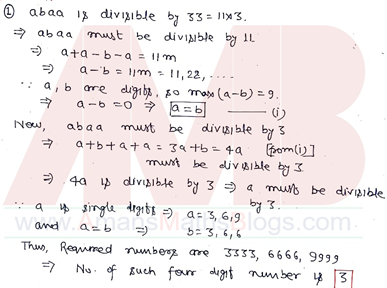Question 2 :

If a + 2a + 3a + … + 1000a = 2b + 4b + 6b + … + 2000b = 3c + 6c + 9c + … + 3000c, then a : b : c is

(A) 1 : 2 : 3

(B) 3 : 2 : 1

(C) 2 : 3 : 6

(D) 6 : 3 : 2

Solution 2 :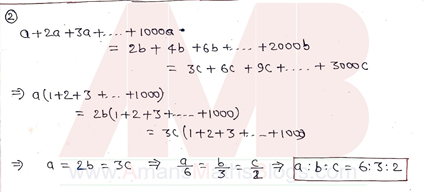### NMTC 2005 Stage 1 Sub Junior Level Questions Paper With Solutions

Question 1 :

You join a job. Your pay for the first day is Rs 5. Each day after that your pay will be twice as much as it was the day before. Your pay on the tenth will be

(A) Rs. 100

(B) Rs. 250

(C) Rs. 5120

(D) Rs. 2560

Solution 1 :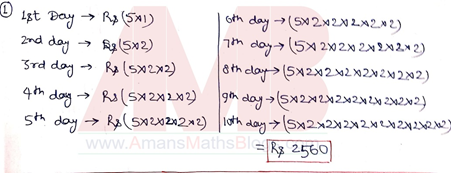Question 2 :

Using all the digits 1 to 9 only one, how many nine digit prime numbers can you write?

(A) 1

(B) 0

(C) 9

(D) More than 100

Solution 2 :### NMTC 2006 Stage 1 Sub Junior Level Questions Paper With Solutions

Question 1 :

Given the alphabetic where each letter represents a different digit. The value of C in the least value for ABCD is(A) 1

(B) 2

(C) 6

(D) 7

Solution 1 :Question 2 :

If 36a4 = a6, then the value of a3 is

(A) (1/6)a6

(B) 6a4

(C) (1/6)a2

(D) 6a2

Solution 2 :### NMTC 2007 Stage 1 Sub Junior Level Questions Paper With Solutions

Question 1 :

a, b, c, d are real numbers such that a – 2005 = b + 2006 = c – 2007 = d + 2008. The greatest among a, b, c, d is

(A) a

(B) b

(C) c

(D) d

Solution 1 :Question 2 :

a, b, c, d are real numbers such that a – 2005 = b + 2006 = c – 2007 = d + 2008. The greatest among a, b, c, d is

(A) a

(B) b

(C) c

(D) d

Solution 2 :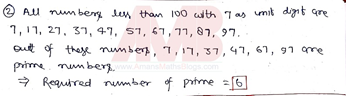### NMTC 2008 Stage 1 Sub Junior Level Questions Paper With Solutions

Question 1 :

In the adjoining figure, l1 and l2 are parallel lines. T is transversal which cuts l1, l2 at A and B respectively. The angles at A, B (refer figure) are trisected. The measure of the angles ACB and ADB are respectively.(A) 60°, 120°

(B) 120°, 60°

(C) x – y, x + y

(D) 2(x – y), (x + y)

Solution 1 :Question 2 :

The number of digits when 2008 is written in the decimal form.

(A) 2008

(B) 1004

(C) 74

(D) 19

Solution 2 :### NMTC 2009 Stage 1 Sub Junior Level Questions Paper With Solutions

Question 1 :

The number of three digit numbers whose digits are even is

(A) 64

(B) 75

(C) 100

(D) 125

Solution 1 :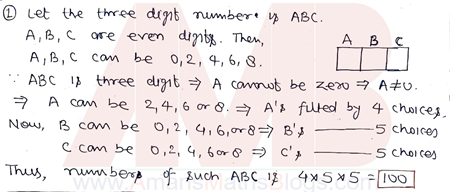Question 2 :

ABCD is a square and BCE is an equilateral triangle constructed externally. The measure of ∠AED is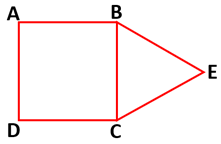(A) 30°

(B) 20°

(C) 35°

(D) 15°

Solution 2 :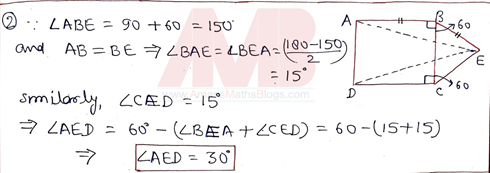### NMTC 2010 Stage 1 Sub Junior Level Questions Paper With Solutions

Question 1 :

Given a sequence of two digit numbers grouped in brackets as follows (10), (11, 20), (12, 21, 30), (13, 23, 31, 40), …(89, 98), (99). The digital sum of the numbers in the brackets having maximum numbers is

(A) 9

(B) 10

(C) 9 or 10

(D) 18

Solution 1 :Question 2 :

Using the digits 2 and 7, and addition or subtraction operations only, the number 2010 is written. The maximum number of 7 that can be used, so that the total numbers used is a minimum is

(A) 284

(B) 286

(C) 288

(D) 290

Solution 2 :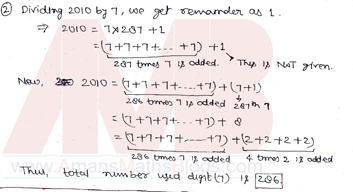### NMTC 2011 Stage 1 Sub Junior Level Questions Paper With Solutions

Question 1 :

Given 63.63 = m(21 + n/100), m, n positive integers with n < 100. The value of (m + n) is

(A) 21

(B) 24

(C) 104

(D) 101

Solution 1 :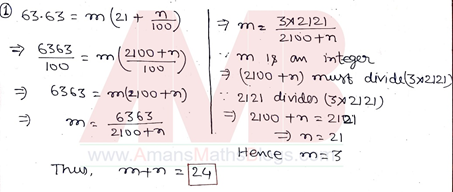Question 2 :

n is a natural number and (n + 2)(n + 4) is odd. Then the biggest power of 2 that divides (n + 1)(n + 3) for any n is

(A) 1

(B) 2

(C) 3

(D) 4

Solution 2 :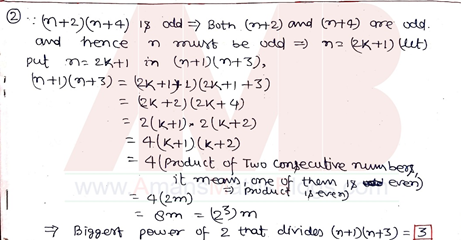### NMTC 2012 Stage 1 Sub Junior Level Questions Paper With Solutions

Question 1 :

p is an odd prime. Thenis

(A) a fraction less than p

(B) a fraction greater than p

(C) a natural number NOT equal to p

(D) a natural number equal to p

Solution 1 :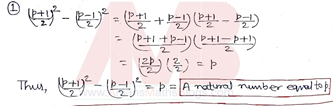Question 2 :

PQRS is a parallelogram. MP and NP divide ∠SPQ into three equal parts (∠MPQ > ∠NPQ) and MQ and NQ divide ∠RQP into three equal parts (∠MQP > ∠NQP). If k(∠PNQ) = (∠PMQ), then k =

(A) 1/2

(B) 1a fraction greater than p

(C) 3/2

(D) 1/3

Solution 2 :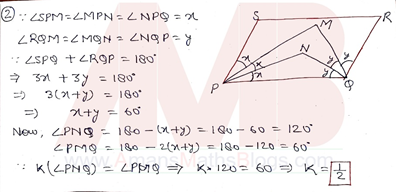### NMTC 2013 Stage 1 Sub Junior Level Questions Paper With Solutions

Question 1 :

In the adjoining incomplete magic square, the sum of all numbers in any row or column or diagonals is a constant value. The value of x is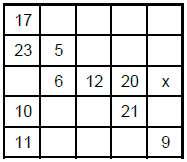(A) 18

(B) 23

(C) 22

(D) 16

Solution 1 :Question 2 :

The sum of three different prime numbers is 40. What is the difference between the two biggest ones among them

(A) 8

(B) 12

(C) 20

(D) 24

Solution 2 :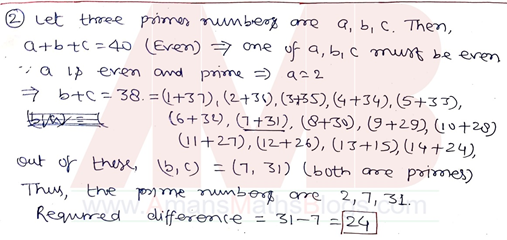### NMTC 2014 Stage 1 Sub Junior Level Questions Paper With Solutions

Question 1 :

If 150% of a certain number is 300, then 30% of the number is

(A) 18

(B) 23

(C) 22

(D) 16

Solution 1 :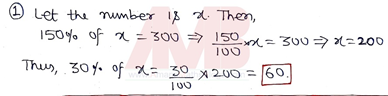Question 2 :

The sum of five distinct non-negative integers is 90. What can be the second largest number of the five at most?

(A) 82

(B) 43

(C) 34

(D) 73

Solution 2 :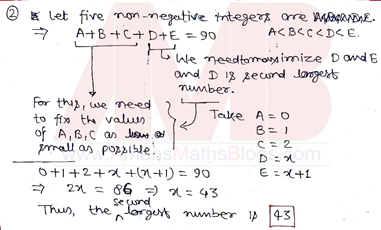### NMTC 2015 Stage 1 Sub Junior Level Questions Paper With Solutions

Question 1 :

The ratio of the angles of a quadrilateral are 7 : 9 : 10 : 10. Then

(A) One angle of the quadrilateral is greater than 120°.

(B) Only one angle of the quadrilateral is 90°.

(C) The sum of some two angles of the quadrilateral is 100°.

(D) There are exactly two right angles as interior angles.

Solution 1 :Question 2 :

Three different integers have a sum 1 and product 36. Then

(A) Certainly all of them are positive

(B) Only one is negative

(C) Exactly two of them are negative

(D) All the three are negative

Solution 2 :### NMTC 2016 Stage 1 Sub Junior Level Questions Paper With Solutions

Question 1 :

On the square ABCD , point E lies on the side AD and F lies on BC so that BE = EF = FD = 30 cm. The area of the square (in square cms) is(A) 300

(B) 900

(C) 800

(D) None of these

Solution 1 :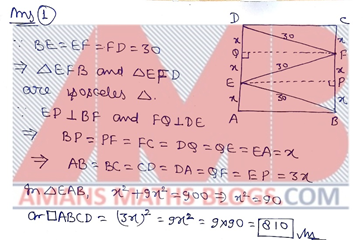Question 2 :

If 2016 = 2x3y5z7u where x, y, z, u are non-negative integers, the value of x + y + 2016z + 3u is

(A) 10

(B) 11

(C) 12

(D) 13

Solution 2 :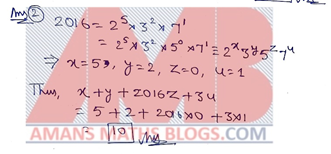### NMTC 2017 Stage 1 Sub Junior Level Questions Paper With Solutions

Question 1 :

The fraction 4/37 is written in the decimal form 0.a1a2a3…. The value of a2017 is

(A) 8

(B) 0

(C) 1

(D) 5

Solution 1 :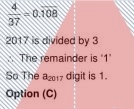Question 2 :

The number of integers x satisfying the equation (x2 – 3x + 1)x + 1 = 1 is

(A) 2

(B) 3

(C) 4

(D) 5

Solution 2 :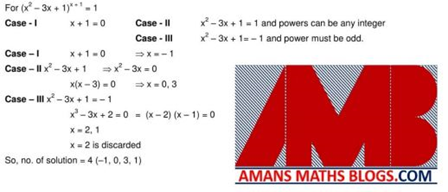### NMTC 2018 Stage 1 Sub Junior Level Questions Paper With Solutions

Question 1 :

The fraction greater than 8 4/9 is

(A) 8 1/3

(B) 150/18

(C) 8 2/3

(D) 216/27

Solution 1 :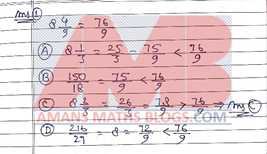Question 2 :

A car is slowly driven in the road full of fog. The car passes a man who was walking at the rate of 3 km an hour in the same direction. He could see the car for 4 minutes and was visible for up to a distance of 100 meters. The speed of the car is (in km per hour)

(A) 8 1/3

(B) 150/18

(C) 8 2/3

(D) 216/27

Solution 2 :### NMTC 2019 Stage 1 Sub Junior Level Questions Paper With Solutions

Question 1 :

If 4921 × D = ABBBD, then the sum of the digits of ABBBD × D is ______.

(A) 19

(B) 20

(C) 25

(D) 26

Solution 1 :Question 2 :

What is the 2019th digit to the right of the decimal point, in the decimal representation of 5/28?

(A) 2

(B) 4

(C) 8

(D) 7

Solution 2 :## NMTC Stage 2 Sub Junior Level Previous Years Questions Papers with Solutions

After clearing NMTC Stage 1 Sub Junior Level exam you need to prepare for NMTC Stage 2 Sub Junior level exam. For this, you need NMTC Stage 2 Sub Junior Level Previous Years Questions Papers with Solutions.

### NMTC 2004 Stage 2 Sub Junior Level Questions Paper With Solutions

Question 1 :

The greatest common divisor of a and 72 is (a, 72) = 24 and the least common multiples of b and 24 is [b, 24] = 72. Find the GCD (a, b) and LCM [a, b] given that a is the smallest three digit number having this property and b is the biggest integer having this property.

Solution 1 :### NMTC 2005 Stage 2 Sub Junior Level Questions Paper With Solutions

Question 1 :

Let N be the greatest integral multiple of 8 such that no two of its digits are same. What is the remainder when N is divided by 1000?

Solution 1 :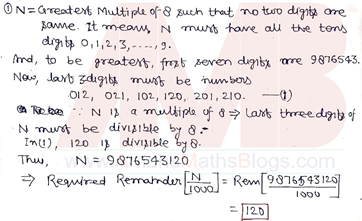### NMTC 2006 Stage 2 Sub Junior Level Questions Paper With Solutions

Question 1 :

If a + 1/a = -1, then find the value of (a2 + a + 1) + (a4 + a2 + 1) + (a6 + a3 + 1) + (a8 + a4 + 1) + … + (a2006 + a1003 + 1).

Solution 1 :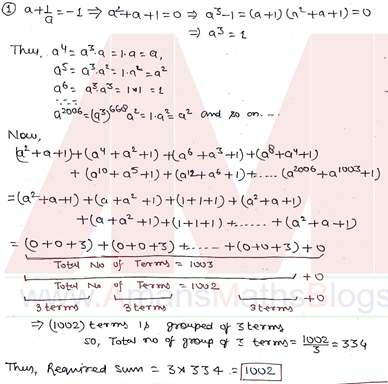### NMTC 2007 Stage 2 Sub Junior Level Questions Paper With Solutions

Question 1 :

The sum of 2000 numbers is 2007. Find the maximum product of these numbers. [There are more than one set of numbers].

Solution 1 :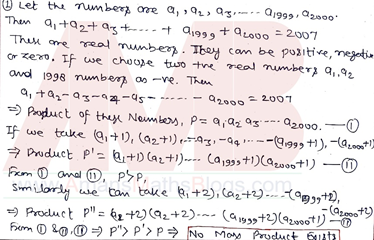### NMTC 2008 Stage 2 Sub Junior Level Questions Paper With Solutions

Question 1 :

A Predator beast weighs 2008 kg at the beginning of a year. During the first month of the year its weighs is increased by 33 1/3% and in the second month decreased by 25% and in the third month increased by 50%, in the fourth month decreased by 33 1/3%, in the fifth month increased by 16 2/3% and in the sixth month decreased by 21 3/7%. This increase and decrease of the weight continued in the next 6 months in the same order with the same percentage. Find the weight of the predator at the end of the year.

Solution 1 :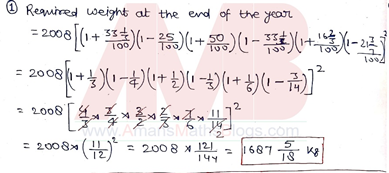### NMTC 2009 Stage 2 Sub Junior Level Questions Paper With Solutions

Question 1 :

Find the number of distinct remainders when 2009 is divided by natural numbers. Note that the remainder r is such that 0 ≤ r < 2009.

Solution 1 :### NMTC 2010 Stage 2 Sub Junior Level Questions Paper With Solutions

Question 1 :

a and b are positive integers with a > b. Find all pairs a, b such that the sum of their sum, difference, product and quotient is 36

Solution 1 :### NMTC 2011 Stage 2 Sub Junior Level Questions Paper With Solutions

Question 1 :

Find all the three digit and four digit natural numbers such that the product of the digits is a prime number. Find the sum of all such three digit numbers and the sum of all such four digit numbers. Find the biggest prime factor of each sum.

Solution 1 :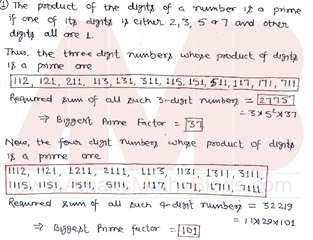### NMTC 2012 Stage 2 Sub Junior Level Questions Paper With Solutions

Question 1 :

Find the number of numbers coprime to and less than 2012. Find their sum. Find also the quotient when this sum is divided by 2012. (Information: 503 is a prime).

Solution 1 :### NMTC 2013 Stage 2 Sub Junior Level Questions Paper With Solutions

Question 2 :

The ratio between two digit number and the sum of the digits of that number is a : b. If the digit in the unit place is n more than the digits in the tens place, prove that the number is given by 9na/(11b – 2a).

Solution 2 :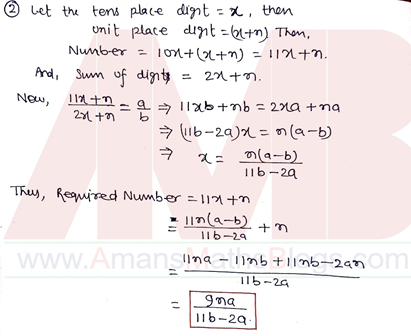### NMTC 2014 Stage 2 Sub Junior Level Questions Paper With Solutions

Question 1 :

The ratio between two digit number and the sum of the digits of that number is a : b. If the digit in the unit place is n more than the digits in the tens place, prove that the number is given by 9na/(11b – 2a).

Solution 1 :### NMTC 2015 Stage 2 Sub Junior Level Questions Paper With Solutions

Question 1 :

The diagram below contains 13 boxes. The numbers in the second and the twelfth boxes are respectively 175 and 70. Fill up in the boxes with natural numbers such that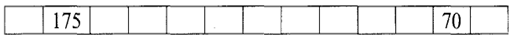• Sum of all numbers in all the 13 boxes is 2015.
• Sum of the numbers in any three consecutive boxes is always the same.

The solution must contain the steps how you arrive at the numbers

Solution 1 :### NMTC 2016 Stage 2 Sub Junior Level Questions Paper With Solutions

Question 1 (a):

If x/a = y/b = z/c = 2016, where x, y, z, a, b, c are non-zero real numbers, find the value ofSolution 1 (a):### NMTC 2017 Stage 2 Sub Junior Level Questions Paper With Solutions

Question 1(a) :

Find all three-digit numbers in which any two adjacent digits differ by 3

Solution 1(a) :### NMTC 2018 Stage 2 Sub Junior Level Questions Paper With Solutions

Question 2 :

In the figure given, ∠A, ∠B and ∠C are right angles. If and ∠AEB = 40° and ∠BED = ∠BDE, then find ∠CDE.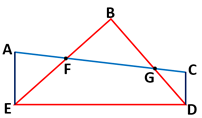Solution 2 :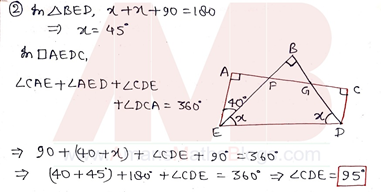### NMTC 2019 Stage 2 Sub Junior Level Questions Paper With Solutions

Question 4 :

In a triangle XYZ, the medians drawn through X and Y are perpendicular. Then, show that XY is the smallest side of the triangle XYZ

Solution 4 :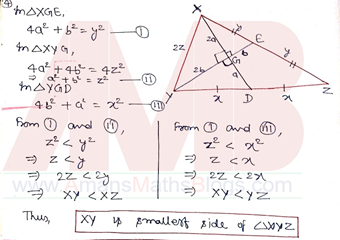## Get NMTC Sub Junior Level Previous Years Papers with Solutions EBook

To get the EBook PDF of NMTC 2004 to 2019 Stage 1 and 2 for Sub junior Level Questions Papers With Answer Keys & Solutions, Click on Image Below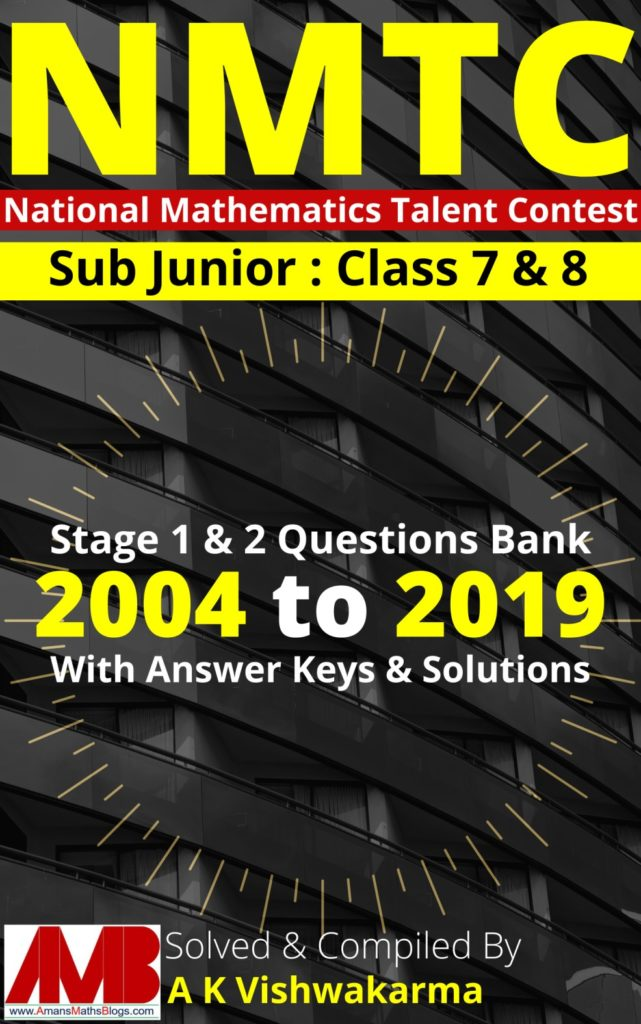PDF File Size : 64 MB Number of Pages : 342

error: Content is protected !!